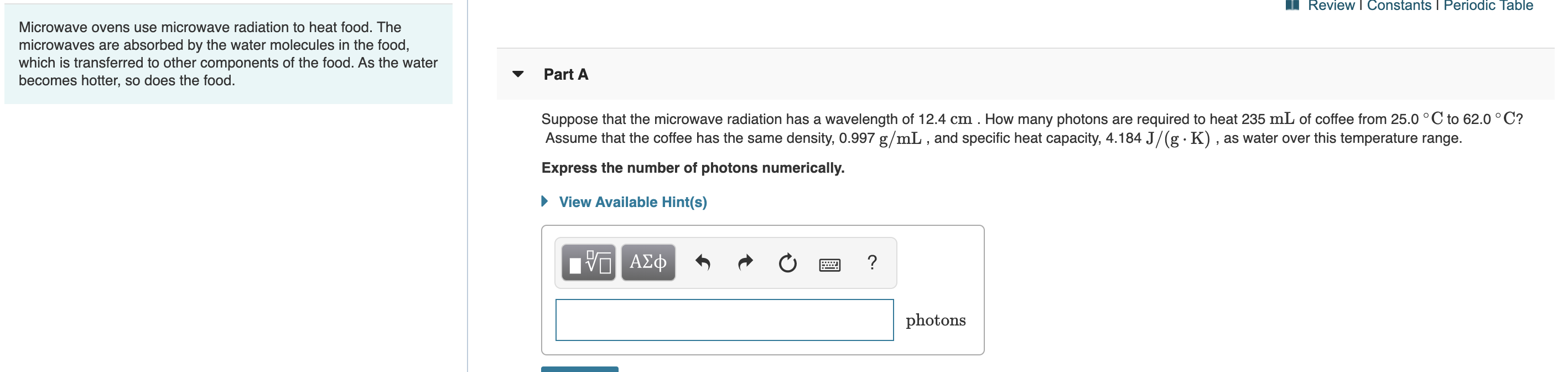# Review I Constants I Periodic TableMicrowave ovens use microwave radiation to heat food. Themicrowaves are absorbed by the water molecules in the food,which is transferred to other components of the food. As the waterbecomes hotter, so does the food.Part ASuppose that the microwave radiation has a wavelength of 12.4 cm . How many photons are required to heat 235 mL of coffee from 25.0 °C to 62.0 °C?Assume that the coffee has the same density, 0.997 g/mL , and specific heat capacity, 4.184 J/(g · K) , as water over this temperature range.Express the number of photons numerically.• View Available Hint(s)Hν ΑΣφphotons

Question
1 viewshelp_outlineImage TranscriptioncloseReview I Constants I Periodic Table Microwave ovens use microwave radiation to heat food. The microwaves are absorbed by the water molecules in the food, which is transferred to other components of the food. As the water becomes hotter, so does the food. Part A Suppose that the microwave radiation has a wavelength of 12.4 cm . How many photons are required to heat 235 mL of coffee from 25.0 °C to 62.0 °C? Assume that the coffee has the same density, 0.997 g/mL , and specific heat capacity, 4.184 J/(g · K) , as water over this temperature range. Express the number of photons numerically. • View Available Hint(s) Hν ΑΣφ photons fullscreen
check_circle

Step 1

Specific heat capacity is 4.184 J/g.K.

Heat applied = ?

Initial temperature = 25oC = 298 K

Final temperature = 62 oC = 335 K

Mass of coffee = volume x density = 235 ml x 0.997 g/ml = 234.295 g

Heat required to increase the temperature can be can be calculated as follows...

### Want to see the full answer?

See Solution

#### Want to see this answer and more?

Solutions are written by subject experts who are available 24/7. Questions are typically answered within 1 hour.*

See Solution
*Response times may vary by subject and question.
Tagged in

### Chemistry